/scikit-learn

sklearn.mixture.GaussianMixture

`class sklearn.mixture.GaussianMixture(n_components=1, covariance_type=’full’, tol=0.001, reg_covar=1e-06, max_iter=100, n_init=1, init_params=’kmeans’, weights_init=None, means_init=None, precisions_init=None, random_state=None, warm_start=False, verbose=0, verbose_interval=10)` [source]

Gaussian Mixture.

Representation of a Gaussian mixture model probability distribution. This class allows to estimate the parameters of a Gaussian mixture distribution.

Read more in the User Guide.

New in version 0.18.

Parameters: `n_components : int, defaults to 1.` The number of mixture components. `covariance_type : {‘full’ (default), ‘tied’, ‘diag’, ‘spherical’}` String describing the type of covariance parameters to use. Must be one of: ‘full’ each component has its own general covariance matrix ‘tied’ all components share the same general covariance matrix ‘diag’ each component has its own diagonal covariance matrix ‘spherical’ each component has its own single variance `tol : float, defaults to 1e-3.` The convergence threshold. EM iterations will stop when the lower bound average gain is below this threshold. `reg_covar : float, defaults to 1e-6.` Non-negative regularization added to the diagonal of covariance. Allows to assure that the covariance matrices are all positive. `max_iter : int, defaults to 100.` The number of EM iterations to perform. `n_init : int, defaults to 1.` The number of initializations to perform. The best results are kept. `init_params : {‘kmeans’, ‘random’}, defaults to ‘kmeans’.` The method used to initialize the weights, the means and the precisions. Must be one of: ```'kmeans' : responsibilities are initialized using kmeans. 'random' : responsibilities are initialized randomly. ``` `weights_init : array-like, shape (n_components, ), optional` The user-provided initial weights, defaults to None. If it None, weights are initialized using the `init_params` method. `means_init : array-like, shape (n_components, n_features), optional` The user-provided initial means, defaults to None, If it None, means are initialized using the `init_params` method. `precisions_init : array-like, optional.` The user-provided initial precisions (inverse of the covariance matrices), defaults to None. If it None, precisions are initialized using the ‘init_params’ method. The shape depends on ‘covariance_type’: ```(n_components,) if 'spherical', (n_features, n_features) if 'tied', (n_components, n_features) if 'diag', (n_components, n_features, n_features) if 'full' ``` `random_state : int, RandomState instance or None, optional (default=None)` If int, random_state is the seed used by the random number generator; If RandomState instance, random_state is the random number generator; If None, the random number generator is the RandomState instance used by `np.random`. `warm_start : bool, default to False.` If ‘warm_start’ is True, the solution of the last fitting is used as initialization for the next call of fit(). This can speed up convergence when fit is called several times on similar problems. In that case, ‘n_init’ is ignored and only a single initialization occurs upon the first call. See the Glossary. `verbose : int, default to 0.` Enable verbose output. If 1 then it prints the current initialization and each iteration step. If greater than 1 then it prints also the log probability and the time needed for each step. `verbose_interval : int, default to 10.` Number of iteration done before the next print. `weights_ : array-like, shape (n_components,)` The weights of each mixture components. `means_ : array-like, shape (n_components, n_features)` The mean of each mixture component. `covariances_ : array-like` The covariance of each mixture component. The shape depends on `covariance_type`: ```(n_components,) if 'spherical', (n_features, n_features) if 'tied', (n_components, n_features) if 'diag', (n_components, n_features, n_features) if 'full' ``` `precisions_ : array-like` The precision matrices for each component in the mixture. A precision matrix is the inverse of a covariance matrix. A covariance matrix is symmetric positive definite so the mixture of Gaussian can be equivalently parameterized by the precision matrices. Storing the precision matrices instead of the covariance matrices makes it more efficient to compute the log-likelihood of new samples at test time. The shape depends on `covariance_type`: ```(n_components,) if 'spherical', (n_features, n_features) if 'tied', (n_components, n_features) if 'diag', (n_components, n_features, n_features) if 'full' ``` `precisions_cholesky_ : array-like` The cholesky decomposition of the precision matrices of each mixture component. A precision matrix is the inverse of a covariance matrix. A covariance matrix is symmetric positive definite so the mixture of Gaussian can be equivalently parameterized by the precision matrices. Storing the precision matrices instead of the covariance matrices makes it more efficient to compute the log-likelihood of new samples at test time. The shape depends on `covariance_type`: ```(n_components,) if 'spherical', (n_features, n_features) if 'tied', (n_components, n_features) if 'diag', (n_components, n_features, n_features) if 'full' ``` `converged_ : bool` True when convergence was reached in fit(), False otherwise. `n_iter_ : int` Number of step used by the best fit of EM to reach the convergence. `lower_bound_ : float` Lower bound value on the log-likelihood (of the training data with respect to the model) of the best fit of EM.

`BayesianGaussianMixture`
Gaussian mixture model fit with a variational inference.

Methods

 `aic`(X) Akaike information criterion for the current model on the input X. `bic`(X) Bayesian information criterion for the current model on the input X. `fit`(X[, y]) Estimate model parameters with the EM algorithm. `fit_predict`(X[, y]) Estimate model parameters using X and predict the labels for X. `get_params`([deep]) Get parameters for this estimator. `predict`(X) Predict the labels for the data samples in X using trained model. `predict_proba`(X) Predict posterior probability of each component given the data. `sample`([n_samples]) Generate random samples from the fitted Gaussian distribution. `score`(X[, y]) Compute the per-sample average log-likelihood of the given data X. `score_samples`(X) Compute the weighted log probabilities for each sample. `set_params`(**params) Set the parameters of this estimator.
`__init__(n_components=1, covariance_type=’full’, tol=0.001, reg_covar=1e-06, max_iter=100, n_init=1, init_params=’kmeans’, weights_init=None, means_init=None, precisions_init=None, random_state=None, warm_start=False, verbose=0, verbose_interval=10)` [source]
`aic(X)` [source]

Akaike information criterion for the current model on the input X.

Parameters: `X : array of shape (n_samples, n_dimensions)` `aic : float` The lower the better.
`bic(X)` [source]

Bayesian information criterion for the current model on the input X.

Parameters: `X : array of shape (n_samples, n_dimensions)` `bic : float` The lower the better.
`fit(X, y=None)` [source]

Estimate model parameters with the EM algorithm.

The method fits the model `n_init` times and sets the parameters with which the model has the largest likelihood or lower bound. Within each trial, the method iterates between E-step and M-step for `max_iter` times until the change of likelihood or lower bound is less than `tol`, otherwise, a `ConvergenceWarning` is raised. If `warm_start` is `True`, then `n_init` is ignored and a single initialization is performed upon the first call. Upon consecutive calls, training starts where it left off.

Parameters: `X : array-like, shape (n_samples, n_features)` List of n_features-dimensional data points. Each row corresponds to a single data point. self
`fit_predict(X, y=None)` [source]

Estimate model parameters using X and predict the labels for X.

The method fits the model n_init times and sets the parameters with which the model has the largest likelihood or lower bound. Within each trial, the method iterates between E-step and M-step for `max_iter` times until the change of likelihood or lower bound is less than `tol`, otherwise, a `ConvergenceWarning` is raised. After fitting, it predicts the most probable label for the input data points.

New in version 0.20.

Parameters: `X : array-like, shape (n_samples, n_features)` List of n_features-dimensional data points. Each row corresponds to a single data point. `labels : array, shape (n_samples,)` Component labels.
`get_params(deep=True)` [source]

Get parameters for this estimator.

Parameters: `deep : boolean, optional` If True, will return the parameters for this estimator and contained subobjects that are estimators. `params : mapping of string to any` Parameter names mapped to their values.
`predict(X)` [source]

Predict the labels for the data samples in X using trained model.

Parameters: `X : array-like, shape (n_samples, n_features)` List of n_features-dimensional data points. Each row corresponds to a single data point. `labels : array, shape (n_samples,)` Component labels.
`predict_proba(X)` [source]

Predict posterior probability of each component given the data.

Parameters: `X : array-like, shape (n_samples, n_features)` List of n_features-dimensional data points. Each row corresponds to a single data point. `resp : array, shape (n_samples, n_components)` Returns the probability each Gaussian (state) in the model given each sample.
`sample(n_samples=1)` [source]

Generate random samples from the fitted Gaussian distribution.

Parameters: `n_samples : int, optional` Number of samples to generate. Defaults to 1. `X : array, shape (n_samples, n_features)` Randomly generated sample `y : array, shape (nsamples,)` Component labels
`score(X, y=None)` [source]

Compute the per-sample average log-likelihood of the given data X.

Parameters: `X : array-like, shape (n_samples, n_dimensions)` List of n_features-dimensional data points. Each row corresponds to a single data point. `log_likelihood : float` Log likelihood of the Gaussian mixture given X.
`score_samples(X)` [source]

Compute the weighted log probabilities for each sample.

Parameters: `X : array-like, shape (n_samples, n_features)` List of n_features-dimensional data points. Each row corresponds to a single data point. `log_prob : array, shape (n_samples,)` Log probabilities of each data point in X.
`set_params(**params)` [source]

Set the parameters of this estimator.

The method works on simple estimators as well as on nested objects (such as pipelines). The latter have parameters of the form `<component>__<parameter>` so that it’s possible to update each component of a nested object.

Returns: self

Examples using `sklearn.mixture.GaussianMixture`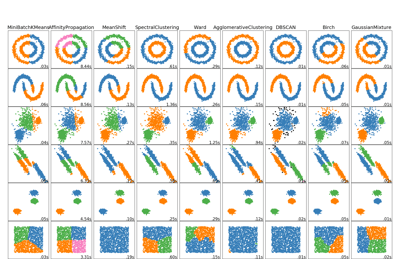Comparing different clustering algorithms on toy datasets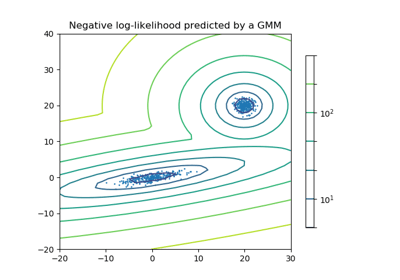Density Estimation for a Gaussian mixture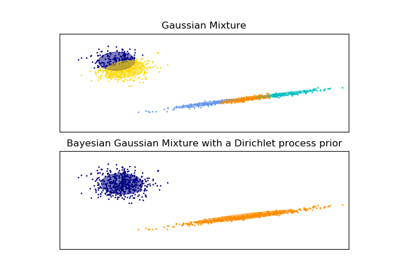Gaussian Mixture Model Ellipsoids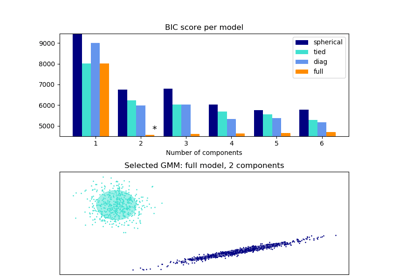Gaussian Mixture Model Selection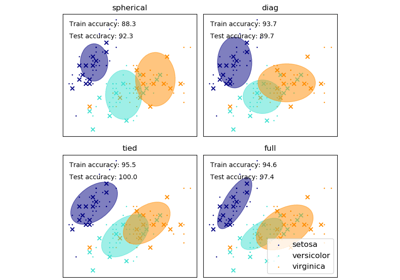GMM covariances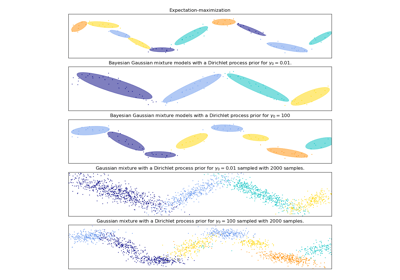Gaussian Mixture Model Sine Curve